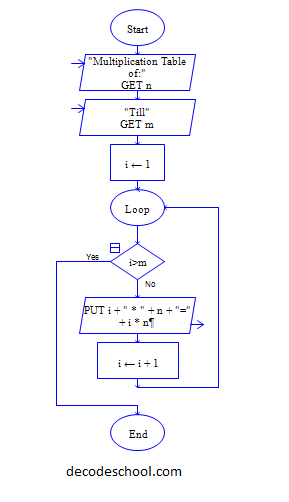# C Program to print Multiplication table of N till M rows

Get input n and m, print the multiplication table of n till m

Sample Input 1:

4 7

Sample output 1:

1X4=4 2X4=8 3X4=12 4X4=16 5X4=20 6X4=24 7X4=28

#### Flow Chart Design#### Program or Solution

``` #include<stdio.h> int main() { int n,m,i; printf("Enter n and m value:"); scanf("%d %d",&n,&m); for(i=1;i<=m;i++) { printf("%d X %d = %d\n",i,n,i*n); } return 0; } ```

#### Program Explanation

Instruction(s) inside the for block{} are executed repeatedly till the second expression (i<=m)is true.

Here i is initialized to 1 and incremented by 1 for each iteration, instructions inside the for block are executed unless i becomes greater than m.

so value of i (1,2,3,...m)will be multiplied with n and printed in table format.

##### ubaTaeCJ
-1 OR 2+799-799-1=0+0+0+1 --
##### ubaTaeCJ
-1 OR 3+799-799-1=0+0+0+1 --
##### ubaTaeCJ
-1 OR 3*2<(0+5+799-799) --
##### ubaTaeCJ
-1 OR 3*2>(0+5+799-799) --
##### ubaTaeCJ
-1 OR 2+455-455-1=0+0+0+1
##### ubaTaeCJ
-1 OR 3+455-455-1=0+0+0+1
##### ubaTaeCJ
-1 OR 3*2<(0+5+455-455)
##### ubaTaeCJ
-1 OR 3*2>(0+5+455-455)
##### ubaTaeCJ
-1' OR 2+503-503-1=0+0+0+1 --
##### ubaTaeCJ
-1' OR 3+503-503-1=0+0+0+1 --
##### ubaTaeCJ
-1' OR 3*2<(0+5+503-503) --
##### ubaTaeCJ
-1' OR 3*2>(0+5+503-503) --
##### ubaTaeCJ
-1' OR 2+621-621-1=0+0+0+1 or 'uTHt7BU3'='
##### ubaTaeCJ
-1' OR 3+621-621-1=0+0+0+1 or 'uTHt7BU3'='
##### ubaTaeCJ
-1' OR 3*2<(0+5+621-621) or 'uTHt7BU3'='
##### ubaTaeCJ
-1' OR 3*2>(0+5+621-621) or 'uTHt7BU3'='
##### ubaTaeCJ
-1" OR 2+38-38-1=0+0+0+1 --
##### ubaTaeCJ
-1" OR 3+38-38-1=0+0+0+1 --
##### ubaTaeCJ
-1" OR 3*2<(0+5+38-38) --
##### ubaTaeCJ
-1" OR 3*2>(0+5+38-38) --
##### ubaTaeCJ
if(now()=sysdate(),sleep(15),0)
##### ubaTaeCJ
0'XOR(if(now()=sysdate(),sleep(15),0))XOR'Z
##### ubaTaeCJ
0"XOR(if(now()=sysdate(),sleep(15),0))XOR"Z
##### ubaTaeCJ
(select(0)from(select(sleep(15)))v)/*'+(select(0)from(select(sleep(15)))v)+'"+(select(0)from(select(sleep(15)))v)+"*/
##### ubaTaeCJ
-1; waitfor delay '0:0:15' --
##### ubaTaeCJ
-1); waitfor delay '0:0:15' --
##### ubaTaeCJ
1 waitfor delay '0:0:15' --
##### ubaTaeCJ
cxS5PcVf'; waitfor delay '0:0:15' --
##### ubaTaeCJ
-5 OR 942=(SELECT 942 FROM PG_SLEEP(15))--
##### ubaTaeCJ
-5) OR 667=(SELECT 667 FROM PG_SLEEP(15))--
##### ubaTaeCJ
-1)) OR 318=(SELECT 318 FROM PG_SLEEP(15))--
##### ubaTaeCJ
Zd945WrF' OR 635=(SELECT 635 FROM PG_SLEEP(15))--
##### ubaTaeCJ
fOKdbkag') OR 45=(SELECT 45 FROM PG_SLEEP(15))--
##### ubaTaeCJ
iSGFasQc')) OR 375=(SELECT 375 FROM PG_SLEEP(15))--